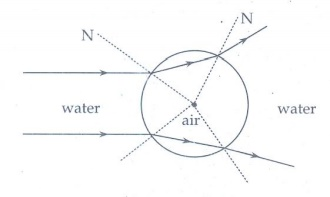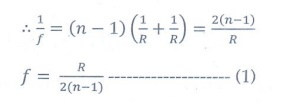Home | | Physics 12th Std | Conceptual Questions

# Conceptual Questions

Physics : Ray Optics: Book Back Important Questions, Answers, Solutions, Conceptual Questions

Conceptual Questions

1. Why are dish antennas curved?

In a dish antenna the curved surface act as a concave mirror. A receiver is placed at the focus of the curved surface.

All the signals which fall on it can be reflected to that receiver. Thereby the strength of signal received is maximum. So the dish antennas are curved.

2. What type of lens is formed by a bubble inside water?

When a ray of light strikes a bubble in water, light ray passes from denser medium (water) to rarer medium (air), it bends away from normal. And again at the other end the light ray will enter from rarer (air) to denser (water), and it bends towards the normal.Thus, the bubble inside the water act as a concave (diverging) lens.

3. It is possible for two lenses to produce zero power?

Yes, It is possible, If one lens is converging lens with some focal length, so power P1 = P. And other lens is diverging lens with same focal length but negative, so power P2 = -P, then the combination of these two lenses will be zero.

4. A biconvex lens has focal length f and intensity of light I passing through it. What will be the focal length and intensity for portions of lenses obtained by cutting it vertically and horizontally as shown in figure?Case-1:

For convex lens

1/f  = (n-1) (1/R1- 1/R2)

If the radius of curvature is equal. Then R1 = R , R2 = -RIntensity is directly proportional to the area of the aperture of the lens receiving the light.

I A

Total area of the aperture is A = π(d/2)2 = πd2 / 4

d is the diameter of the aperture.

Case-2:

When cut vertically, R1 = R , R2 = ∞When cut vertically, the focal length is doubled.

In this case, the diameter of the aperature remains same. Therefore the intensity also remains same. I' = I

Case-3:

When cut horizontally, the radius of curvatures remains same. Therefore the focal length also remains same.

f ' = f

In this case, the diameter of the aperature becomes half.The intensity reduce 1/4 times

5. Why is yellow light preferred to during fog?

During fog, it is hard to see from naked eyes. So we use lights in the vehicles.

The fog lights to be single colour, because the different wavelengths of visible light scattered by the fog droplets differently. This is called dispersion.

Due to dispersion, the visibility will decreases for the drivers. So we should use single colour (yellow) light in fog.

The yellow colour has a longer wavelength. So the Rayleigh scattering by the fog is less. Thereby the visibility will increases.

Thus to reduce the dispersion and Rayleigh scattering, we use yellow lights in the vehicles. Thereby we will increase the visibility for the drivers.

Tags : with solution and answers | Ray Optics | Physics , 12th Physics : UNIT 6 : Ray Optics
Study Material, Lecturing Notes, Assignment, Reference, Wiki description explanation, brief detail
12th Physics : UNIT 6 : Ray Optics : Conceptual Questions | with solution and answers | Ray Optics | Physics